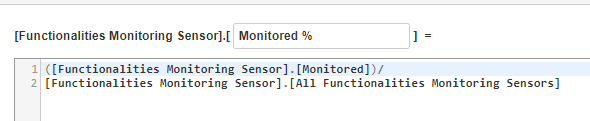# Issue calculating percentage from Insight data

I’m trying to calculate a percentage in Insight data but consistently get 0 out of the division.

For objects created the calculation works (see right side) but I need the SUM of all objects in that status during that month (left side). The numbers are correct are correct.

This is the formula I use.If I select only [Functionalities].[Monitored] it shows the data from the table, if I put in the formula [ Functionalities].[All monitored] it shows the total. However if I do the division I get 0.We usually recommend creating such percentage calculations as additional calculated measures in the “Measures” dimension.

Try the following formula when creating a new calculated measure using integer % format

``````(
[Measures].[Objects in transitions status],
)
/
(
[Measures].[Objects in transitions status],
[Functionalities Monitoring Sensor].DefaultMember
)
``````

After that, you could explore our “drill” feature to show one measure by other dimension members.
see more about that feature here:
https://docs.eazybi.com/eazybijira/analyze-and-visualize/create-reports#Createreports-Drillintomeasurebyanotherdimensionlevel

Martins / eazyBI support

Hi Martins,
Thanks for the tip.
I’ve created a measure with formula:
([Functionalities Monitoring Sensor].[Monitored],[Measures].[Objects in transition status])/
[Measures].[Objects in transition status]
and it works.

1 Like